Solid geometry, stereometry - page 23

Solid geometry is the name for the geometry of three-dimensional Euclidean space.

Stereometry deals with the measurements of volumes of various solid figures (three-dimensional figures) including pyramids, prisms and other polyhedrons; cylinders; cones; truncated cones; and balls bounded by spheres.

1. BoxCalculate the angle between box base 9 x 14 and body diagonal length 18.
2. Tank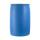In the middle of a cylindrical tank with a bottom diameter 251 cm is standing rod which is 13 cm above the water surface. If we bank rod its end reach surface of the water just by the tank wall. How deep is the tank?
3. Triangular prismBase of perpendicular triangular prism is a right triangle with leg length 5 cm. Content area of the largest side wall of its surface is 130 cm² and the height of the body is 10 cm. Calculate its volume.
4. Axial sectionAxial section of the cylinder has a diagonal 40 cm. The size of the shell and the base surface are in the ratio 3:2. Calculate the volume and surface area of this cylinder.
5. Felix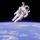Calculate how much land saw Felix Baumgartner after jump from 32 km above ground. The radius of the Earth is R = 6378 km.
6. Equilateral cylinderEquilateral cylinder (height = base diameter; h = 2r) has a volume of V = 199 cm3 . Calculate the surface area of the cylinder.
7. CuboidCuboid ABCDEFGH with 10 cm height has a base edge length 6 cm and 8 cm. Determine angle between body diagonal and the base plane (round to degrees).
8. Children poolThe bottom of the children's pool is a regular hexagon with a = 60 cm side. The distance of opposing sides is 104 cm, the height of the pool is 45 cm. A) How many liters of water can fit into the pool? B) The pool is made of a double layer of plastic film.
9. Tetrahedral prismCalculate surface and volume tetrahedral prism, which has a rhomboid-shaped base, and its dimensions are: a = 12 cm, b = 7 cm, ha = 6 cm and prism height h = 10 cm.
10. Horizon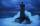The top of a lighthouse is 17 m above the sea. How far away is an object which is just “on the horizon”? [Assume the earth is a sphere of radius 6378.1 km.]
11. Rhombus baseCalculate the volume and surface area of prisms whose base is a rhombus with diagonals u1 = 12 cm and u2 = 10 cm. Prism height is twice base edge length.
12. Truncated pyramidHow many cubic meters is volume of a regular four-side truncated pyramid with edges one meter and 60 cm and high 250 mm?
13. Pine woodFrom a trunk of pine 6m long and 35 cm in diameter with a carved beam with a cross-section in the shape of a square so that the square had the greatest content area. Calculate the length of the sides of a square. Calculate the volume in cubic meters of lum
14. Nice prismCalculate the surface of the cuboid if the sum of its edges is a + b + c = 19 cm and the body diagonal size u = 13 cm.
15. Concrete pipe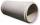Concrete pipe is cylindrical with an inner diameter 110 cm and outer 120 cm. Calculate the surface of the concrete pipe, if it is 9 m long.
16. Sphere - partsCalculate the area of a spherical cap, which is part of an area with base radius ρ = 9 cm and a height v = 3.1 cm.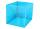I have homework. The cube has an edge 7 cm long and I must find wall and body diagonal.
18. Horizontal Cylindrical SegmentHow much fuel is in the tank of horizontal cylindrical segment with a length 10m, width of level 1 meter and level is 0.2 meters below the upper side of the tank?
19. Angle of deviationThe surface of the rotating cone is 30 cm2 (with circle base), its surface area is 20 cm2. Calculate the deviation of the side of this cone from the plane of the base.
20. VintnerHow high can vintner fill keg with crushed red grapes if these grapes occupy a volume of 20 percent? Keg is cylindrical with a diameter of the base 1 m and a volume 9.42 hl. Start from the premise that says that fermentation will fill the keg (the number.

Do you have an interesting mathematical example that you can't solve it? Enter it, and we can try to solve it.

To this e-mail address, we will reply solution; solved examples are also published here. Please enter e-mail correctly and check whether you don't have a full mailbox.